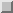Next:
IANY_PREFIX(ARRAYDIM, MASK, Up: Specifications of Library Previous: IALL_SUFFIX(ARRAYDIM, MASK,

## IANY(ARRAY, DIM, MASK)

Optional Arguments. DIM, MASK
Description. Computes a bitwise logical OR reduction along dimension DIM of ARRAY.
Class. Transformational function.
Arguments.

ARRAY must be of type integer. It must not be scalar.

DIM (optional) must be scalar and of type integer with a value in the range, whereis the rank of ARRAY. The corresponding actual argument must not be an optional dummy argument.

MASK (optional) must be of type logical and must be conformable with ARRAY.
Result Type, Type Parameter, and Shape. The result is of type integer with the same kind type parameter as ARRAY. It is scalar if DIM is absent or if ARRAY has rank one; otherwise, the result is an array of rankand shapewhereis the shape of ARRAY.
Result Value.

(i): The result of IANY(ARRAY) is the IOR reduction of all the elements of ARRAY. If ARRAY has size zero, the result has the value zero. See Section.

(ii): The result of IANY(ARRAY, MASK=MASK) is the IOR reduction of all the elements of ARRAY corresponding to the true elements of MASK; if MASK contains no true elements, the result is zero.

(iii): If ARRAY has rank one, IANY(ARRAY, DIM [,MASK]) has a value equal to that of IANY(ARRAY [,MASK]). Otherwise, the value of elementof IANY(ARRAY, DIM [,MASK]) is equal to IANY(ARRAY() [,MASK = MASK()])
Examples.

(i): The value of IANY((/9, 8, 3, 2/)) is 11. (ii): The value of IANY(C, MASK = BTEST(C,0)) is the IOR reduction of the odd elements of C.

(iii): If B is the array , 2 3 5 0 4 2 ,, plus 1filll -1; 2 3 5 0 4 2 ,; plus 1filll -1: 2 3 5 0 4 2 ,: plus 1filll -1.plus 1filll 2 3 5 0 4 2 ,.-50 2 3 5 0 4 2 , plus 1filll-1, then IANY(B, DIM = 1) is ,2, 7, 7,, plus 1filll -1;2, 7, 7,; plus 1filll -1:2, 7, 7,: plus 1filll -1.2, 7, 7,. plus 1filll -12, 7, 7, plus 1filll-1 and IANY(B, DIM = 2) is ,7, 6,, plus 1filll -1;7, 6,; plus 1filll -1:7, 6,: plus 1filll -1.7, 6,. plus 1filll -17, 6, plus 1filll-1.Next:
IANY_PREFIX(ARRAYDIM, MASK, Up: Specifications of Library Previous: IALL_SUFFIX(ARRAYDIM, MASK,

paula@erc.msstate.edu
Thu Dec 8 16:17:11 CST 1994